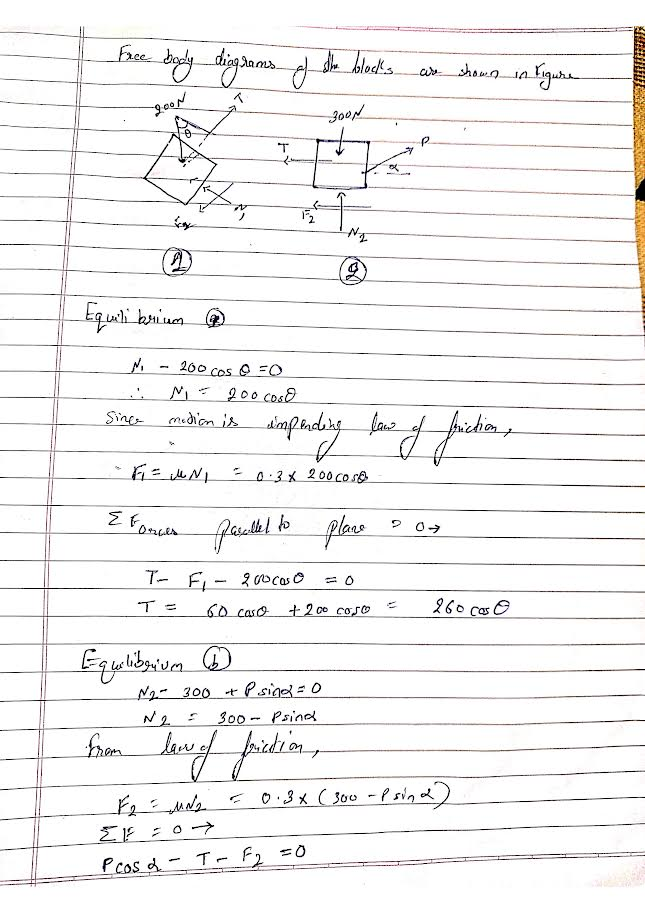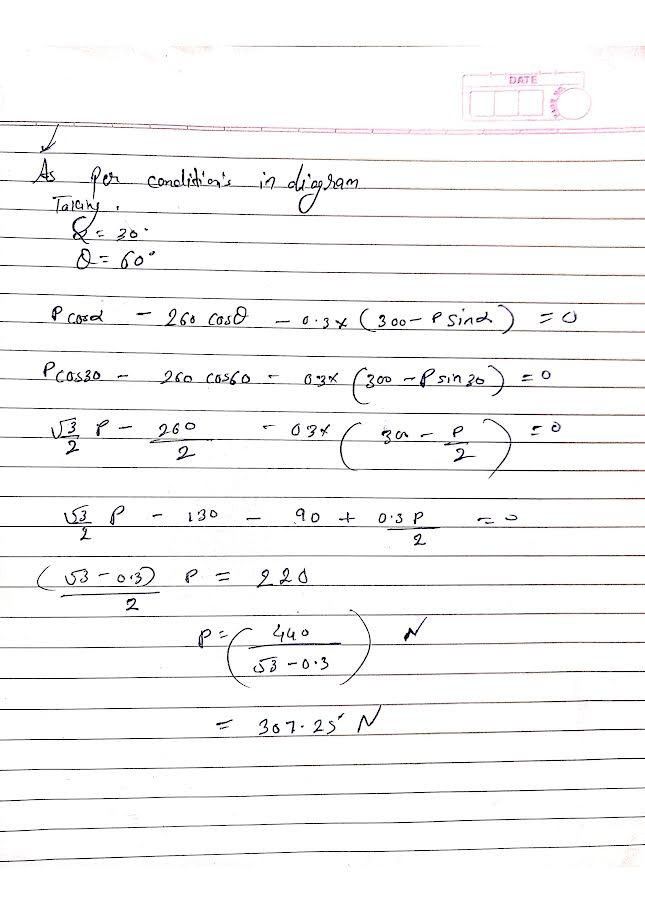# Find the least force P that will just start the system of blocks moving to the right , Take u=0.3 , Assume smooth Pulley

more_vertFind the least force P that will just start the system of blocks moving to the right , Take u=0.3 , Assume smooth Pulley

more_vert

is that angle alpha and theta ? what is 3 are they showing a right angle triangle if yes then alpha=30 and theta=60